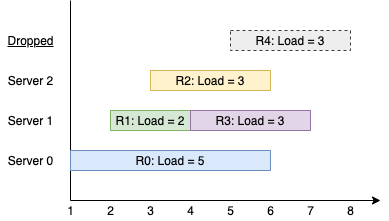| English | 简体中文 |

# 1606. 找到处理最多请求的服务器

## 题目描述

• 第 i （序号从 0 开始）个请求到达。
• 如果所有服务器都已被占据，那么该请求被舍弃（完全不处理）。
• 如果第 (i % k) 个服务器空闲，那么对应服务器会处理该请求。
• 否则，将请求安排给下一个空闲的服务器（服务器构成一个环，必要的话可能从第 0 个服务器开始继续找下一个空闲的服务器）。比方说，如果第 i 个服务器在忙，那么会查看第 (i+1) 个服务器，第 (i+2) 个服务器等等。输入：k = 3, arrival = [1,2,3,4,5], load = [5,2,3,3,3]



输入：k = 3, arrival = [1,2,3,4], load = [1,2,1,2]



输入：k = 3, arrival = [1,2,3], load = [10,12,11]



输入：k = 3, arrival = [1,2,3,4,8,9,10], load = [5,2,10,3,1,2,2]



输入：k = 1, arrival = , load = 



• 1 <= k <= 105
• 1 <= arrival.length, load.length <= 105
• arrival.length == load.length
• 1 <= arrival[i], load[i] <= 109
• arrival 保证 严格递增 。